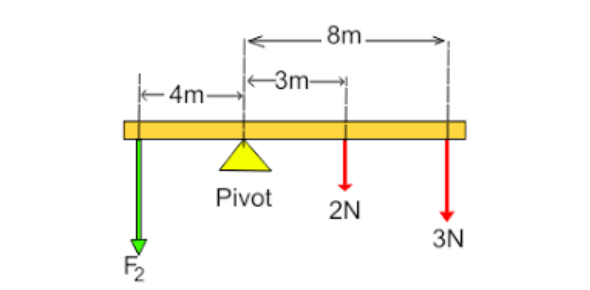# Physics Test: Practice Questions On Moments, Levers And Gears!

10 Questions | Attempts: 10903SettingsIn this "Physics Test: Practice Questions On Moments, Levers, And Gears!" on the scientific topic of physics, we'll be taking a look at the moment of force – which is defined as the measure of its tendency to cause a body to rotate about a specific point of axis. One example you might find easiest to wrap your head around is the see-saw. Think of what causes the see-saw to balance and what tilts it to either side. What do you know about these moments? Take this quiz and find out.

• 1.
For a see-saw to balance, the anti-clockwise moment must equal the clockwise moment. A moment is another word for a turning effect. A see-saw is like a lever, turning around a pivot, also called a fulcrum. A lever is a simple machine which amplifies the effort force.
• A.

True

• B.

False

• 2.
Another name for a pivot is a
• A.

Falcon

• B.

Tool

• C.

Fulcrum

• D.

None of these

• 3.
For a see-saw to balance, the anti-clockwise  moment must equal the ________________
• A.

Clockwise Moment

• B.

Weight

• C.

Mass

• D.

None

• 4.
Moment is the
• A.

Turning effect of a force

• B.

Airflow

• C.

Mass

• D.

Weight

• 5.
What's the unit of the moment?
• A.

Km

• B.

Nk

• C.

Kn

• D.

Nm

• 6.
Newtons (N) is the unit of force.
• A.

True

• B.

False

• 7.
Newtons (K) is the unit of weight.
• A.

True

• B.

False

• 8.
A SEE-SAW. On one side, there is a person with a weight of 600N. He is sitting 3m away from the pivot. On the other side is a person weighing 450N. How far away must (s)he sit?
• A.

2m

• B.

4m

• C.

6m

• D.

3.5m

• E.

3m

• 9.
The force applied to a lever is called
• A.

Force

• B.

Mass

• C.

Effort

• D.

None

## Related TopicsBack to top
×

Wait!
Here's an interesting quiz for you.